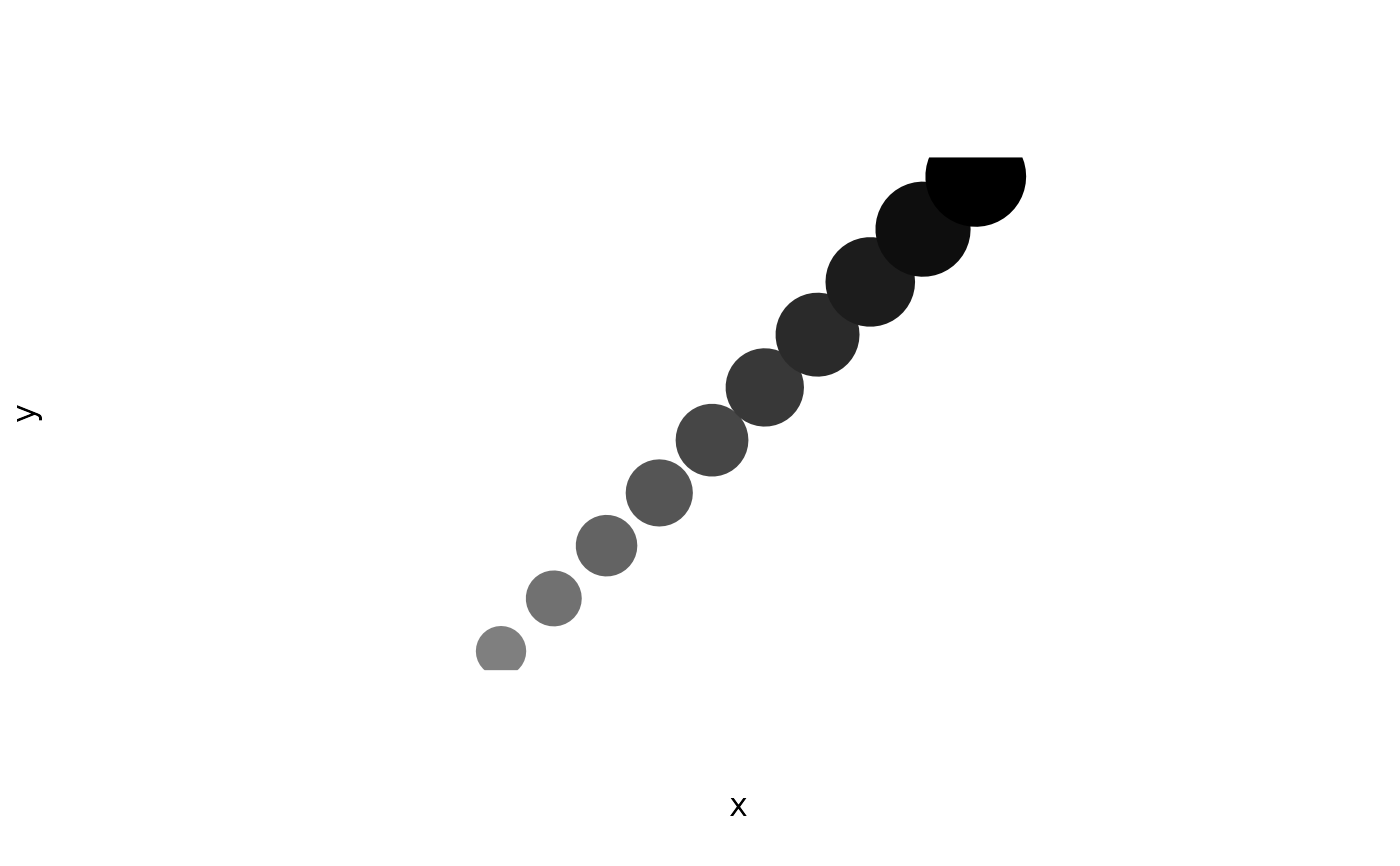Plot3() is an experimental function that generates a two-dimensional plot with the impression of a third dimension obtained through point scaling, overlap and fogging.

Plot3(
x,
y = NULL,
z = NULL,
pch = par("pch"),
col = par("col"),
bg = NA,
cex = 1,
axes = TRUE,
frame.plot = axes,
plot.bg = NA,
fog = 1/2,
shrink = 1/2,
...
)

## Arguments

x, y, z

Coordinates of points to plot.

bg, cex, col, pch, add, axes, frame.plot, ...

Parameters passed to plot.default().

plot.bg

Colour with which to fill plot area, used as fog colour.

fog

Numeric specifying amount of mist to apply to distant points.

shrink

Numeric specifying degree to which size of plotted point should reflect z position.

## Examples

Plot3(1:10, 1:10, 1:10, cex = 7, pch = 16, axes = FALSE, asp = 1)